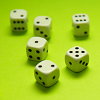#### You may also like### Consecutive Numbers

An investigation involving adding and subtracting sets of consecutive numbers. Lots to find out, lots to explore.### Roll These Dice

Roll two red dice and a green dice. Add the two numbers on the red dice and take away the number on the green. What are all the different possible answers?### Domino Square

Use the 'double-3 down' dominoes to make a square so that each side has eight dots.

# How Do You Do It?

##### Age 7 to 11Challenge Level

This problem encouraged you to work as a group, discussing and sharing different calculation strategies. There may be several different ways of working out the answer to a particular calculation; some are more efficient than others. Can you find different ways of working out the answer to the questions? Look at different types of questions, such as the ones given in the problem. Are the same methods always the most efficient?

William and Conner from St Patrick's R.C. Primary School submitted their solution:

1. answer is $456$ because
\begin{align}20\times24&=480\\480-24&=456\end{align}
2. answer is $1195$ because
\begin{align}200+997&=1197\\1197-2&=1195\end{align}
3. answer is $28.8$ because
\begin{align}57\div2&=28.5\\0.6\div2&=0.3\\0.3+28.5&=28.8\end{align}
4. answer is $3175.7$ because
\begin{align}3841-600&=3241\\3241-65&=3176\\3176-0.3&=3175.7\end{align}
5. answer is $1.3$ because
\begin{align}4\div4&=1\\1.2\div4&=0.3\\1+0.3&=1.3\end{align}
6. answer is $6464$ because
\begin{align}101\times16&=1616\\1600\times4&=6400\\16\times4&=64\\6400+64&=6464\end{align}

William and Conner tackled these problems by splitting the numbers into more familiar-and easier to handle- numbers. They then dealt with these separately and combined the answers.

I wonder whether you used the same methods as William and Conner for each calculation?

Ben, Aeron, Isla and Emily School from St Mary Redcliffe Primary explained how they worked out their answers to the calculations on the cards:

$21\times44$
The easiest way to understand is to add $21$ together $44$ times, but this isn't very quick.
A quick way is to add: $20\times40$, $20\times4$, $1\times40$ and $1\times4$
This is $800+80+40+4 = 924$

$397+998$
We think that the best way is to add the hundreds and then the tens and then the units, and then add them all together.
$300+900 = 1200$, $90+ 90 = 180$, $7+ 8 = 15$
Adding these all together gives $1200+180+15 = 1395$

Half of $31.4$
Half of $30$ is $15$
Half of $1$ is $0.5$
Half of $0.4$ is $0.2$
Adding these $15.7$

$7841 - 239.7$
We thought that the easiest thing to do was to ignore the $7000$, since $841$ is bigger than $249.7$.
So starting with $841$ we took away the two hundred and then the thirty and then the $9$ and then the $0.7$.
So $841$ take away $200$ = $641$
$641$ take away $30$ = $611$
$611$ take away $9$ = $602$
$602$ take away $0.7$ = $601.3$

$7.2$ divided by $5$
To divide by $5$, we divide by ten and then double it.
$7.2$ divided by ten is $0.72$ and doubling that gives $1.44$

$17 \times 102 \times 3$
$17 \times 102$ is the same as $20 \times 102$ take away $3 \times 102$.
This is $1734$.
$1734 \times 3$ is $5202$

The Maths Group at St Teresa's in Surrey had some slightly different methods:

We did the practice calculations in pairs, then presented our methods to the class and voted for the best method in each case. Then we applied them to the new set of calcuations, as follows:

$21 \times 44$:
$10 \times 44 = 440$
Double $440$ = $880$
Add $44$, by adding $20$ and then $24$ = $924$

$397+998$:
Round both: $400 + 1000 = 1400$.
Subtract $5$ = $1395$

Half of $31.4$:
Half of $30$ = $15$
Half of $1.4$ = $0.7$
Add $15 + 0.7 = 15.7$

$7841 - 239.7$
We tried rounding $239.7$ up to $240$, which made the subtraction easy, but we ran into problems later about how much to adjust by!
So, we went:
$800 - 200 = 600$
$40 - 39 = 1$
$1 - 0.7 = 0.3$ and added them up to get $7601.3$

$7.2$ divided by $5$:
we divided by $10$ and got $0.72$ and then doubled it = $1.44$

$17 \times 102 \times 3$:
$17 \times 3 = (10 \times 3) + (7 \times 3) = 51$
$100 \times 51 = 5100$
$2 \times 51 = 102$
Add them up and you get $5202$.

Very well done. I wonder which method you prefer in each case? Or perhaps you have a method that you think works even better for you?